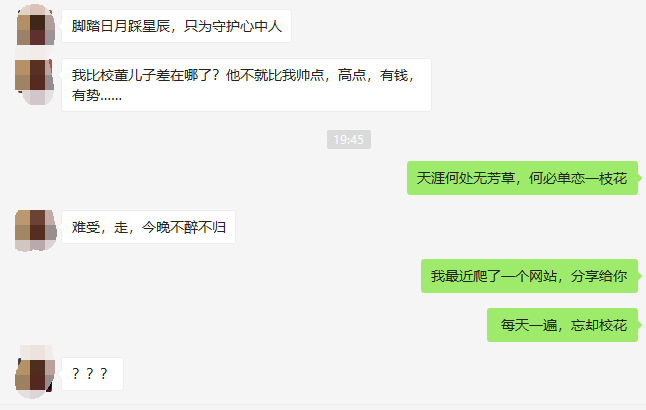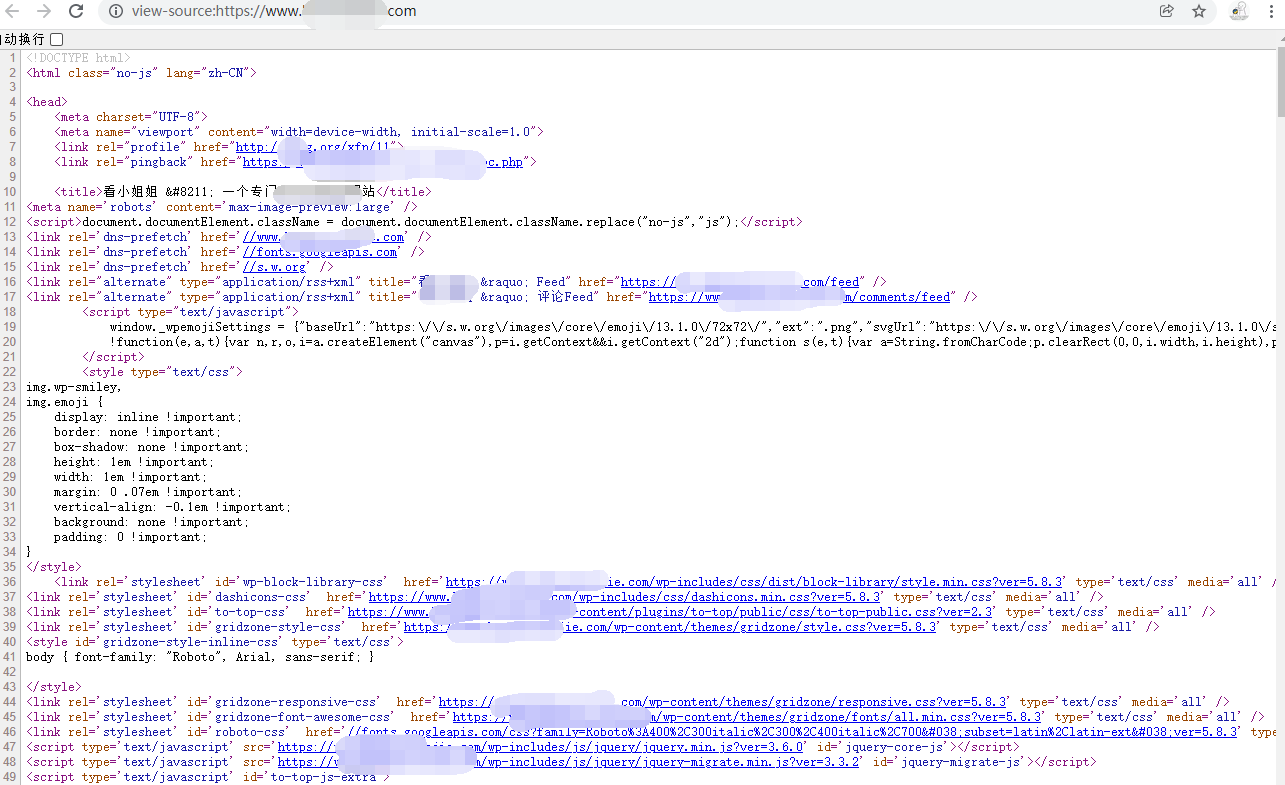# Python里的dict和set的背后小秘密

Python67
• Python里的dict和set的效率有多高?

• 为什么它们是无序的?

• 为什么并不是所有的Python对象都可以当作dict的键或set里的元素?

• 为什么dict的键和set的元素的顺序是根据它们被添加的次序而定的,以及为什么在映射对象的生命周期中,这个顺序并不是一成不变的?

• 为什么不应该在迭代循环dict或是set的同时往里添加元素?

Python里的dict和set的效率有多高?

Python会设法保证大概还有三分之一的表元是空的,所以在快要达到这个阈值的时候,原有散列表会被复制到一个更大的空间里面.

1.散列值和相等性

``````"""
import sys

# 通过sys.maxsize获取操作系统的整数最大值,转换成二进制,计算位数,加上一个符号位
MAX_BITS = len(format(sys.maxsize, 'b'))
print('%s-bit Python build' % (MAX_BITS + 1))

def hash_diff(o1, o2):
h1 = '{:>0{}b}'.format(hash(o1), MAX_BITS)  # 计算o1的散列值,并用0补满空位
h2 = '{:>0{}b}'.format(hash(o2), MAX_BITS)  # 计算o2的散列值,并用0补满空位
# 比较h1和h2的每一位,用!标识出来,否则用' '表示
diff = ''.join('!' if b1 != b2 else ' ' for b1, b2 in zip(h1, h2))
count = '!={}'.format(diff.count('!'))  # 显示不同的总数
width = max(len(repr(o1)), len(repr(o2)), 8)  # 行头的宽度
sep = '_' * (width * 2 + MAX_BITS)  # 分割线
return '{!r:{width}} {}\n{:{width}} {} {}\n{!r:{width}} {}\n{}'.format(
o1, h1, ' ' * width, diff, count, o2, h2, sep, width=width
)

print(hash_diff(1, 1.0))
print(hash_diff(1.0, 1.0001))
print(hash_diff(1.0001, 1.0002))
print(hash_diff(1.0002, 1.0003))
``````

``````|-------------------------------------------------------------------------|
|计算键的散列值               ________使用散列值的另一部分来定位散列表中的零一行 |
|     |                    |                        ↑                     |
|     |                    |                        | 否 (散列冲突)        |
|     |                    ↓                        |                     |
|使用散列值的一部分         表元                       |                     |
|来定位散列表中的一 ------→ 为空? ---------否-------→ 键相等?                 |
|个表元                     |                        |                     |
|                          |是                       |是                   |
|                          ↓                         ↓                     |
|                    抛出KeyError                返回表元里的值              |
|--------------------------------------------------------------------------|
``````

1.键必须死可散列的

1)支持hash()函数,并且通过 `__hash__()`方法所得到的散列值是不变的.

2)支持通过 `__eq__()`方法来检测相等性.

3)若 `a == b`为真,则 `hash(a) == hash(b)`也为真

``````'''

'''
class A:
def __init__(self, a):
self.a = a

def __hash__(self):
return 1

def __eq__(self, other):
return hash_diff(self, other)

def __repr__(self):
return str(self.a)

a = A(1)
b = A(2)
d1 = {a: 1, b: 2, 1: 3}
print(d1)  # {1: 3}  会发现里面只有一个键值对
``````

2.字典在内存上的开销巨大

3.键查询很快

dict的实现是典型的空间换时间:字典类型有着巨大的内存开销,但它们提供了无视数据量的快速访问--只要字典能被装在内存里.

4.键的次序取决于添加顺序

`dict([(key1, value1), (key2, value2)])``dict([(key2, value2), (key1, value1)])`得到的两个字典,在进行比较的时候,它们是相等的.

``````STUDENTS = [
(89, '孙悟空'),
(79, '猪八戒'),
(69, '沙和尚'),
(59, '小白龙'),
(49, '唐僧')
]

d1 = dict(STUDENTS)
print('d1:', d1.keys())
d2 = dict(sorted(STUDENTS))
print('d2:', d2.keys())
d3 = dict(sorted(STUDENTS, key=lambda x: x))
print('d3', d3.keys())
assert d1 == d2 and d2 == d3
``````

5.往字典里添加新键可能会改变已有键的顺序

1.集合里的元素必须是可散列的.

2.集合很消耗内存.

3.可以很高效的判断元素是否存在于某个集合.

4.元素的次序取决于被添加到集合里的次序.

5.往集合里添加元素,可能会改变集合里已有元素的次序.

Original: https://www.cnblogs.com/djdjdj123/p/15483539.html
Author: Python探索牛
Title: Python里的dict和set的背后小秘密

## Title: 死党暗恋校花失败，我爬了这个网站发给他分分钟治愈，男人的快乐往往很简单（每天一遍，忘却初恋）``````python 3.8
pycharm 2021专业版
``````

``````requests
parsel
````````````import requests
import parsel
import re
import os

for page in range(1, 11):
print(f'==================正在爬取第{page}页==================')
# 1.向目标网站发送请求（get，post）
response = requests.get(f'https://www.网站不提供，想用来实践技术的话可以私我拿.com/page/{page}')
# 2. 获取数据（网页源代码）
data_html = response.text
# 3. 解析网页（re正则表达式，css选择器，xpath，bs4，json） 提取每一个详情页的链接与标题
zip_data = re.findall('(.*?)', data_html)
for url, title in zip_data:
print(f'----------------正在爬取{title}----------------')
if not os.path.exists('img/' + title):
os.mkdir('img/' + title)
# 4. 向详情页发送请求
resp = requests.get(url)
# 5. 获取数据（网页源代码）
url_data = resp.text
# 6. 解析网页 （提取图片链接）
selector = parsel.Selector(url_data)
img_list = selector.css('p>img::attr(src)').getall()

for img in img_list:
# 7. 向图片链接发送请求
# 8. 获取数据（图片二进制数据）
img_data = requests.get(img).content
# 9. 保存数据
img_name = img.split('/')[-1]
with open(f"img/{title}/{img_name}", mode='wb') as f:
f.write(img_data)
print(img_name, '爬取成功！！！')
print(title,'爬取成功！！！')
``````
``````#兄弟们学习python，有时候不知道怎么学，从哪里开始学。掌握了基本的一些语法或者做了两个案例后，不知道下一步怎么走，不知道如何去学习更加高深的知识。
#那么对于这些大兄弟们，我准备了大量的免费视频教程，PDF电子书籍，以及视频源的源代码！
#还会有大佬解答！
#都在这个群里了 924040232
#欢迎加入，一起讨论 一起学习！
``````

Original: https://www.cnblogs.com/hahaa/p/15883323.html
Author: 轻松学Python
Title: 死党暗恋校花失败，我爬了这个网站发给他分分钟治愈，男人的快乐往往很简单（每天一遍，忘却初恋）